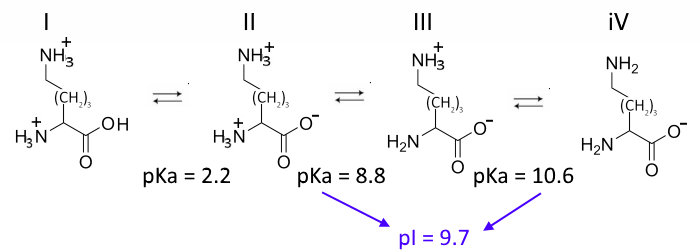Search:

# Constants of acidity and isoelectric point of amino acids

We find the isoelectric point by averaging the $pK_a$ of the different pairs formed by an amino acid:

## Example 1: GlycineDemonstration: Since species II is neutral and I and III have opposite signs, it is necessary to find the pH for which [I] = [III]

(1) $\frac{[H_3O^+][II]}{[I]}=10^{-2.4}$ (2) $\frac{[H_3O^+][III]}{[II]}=10^{-9.6}$ (3) $[III]=[I]$ By multiplying (1) by (2) and introducing (3), we find: $[H_3O^+]^2= 10^{-12}$, hence the result!

## Example 2: Aspartic acidDemonstration: At $pH = 2.9$ , species IV has a negligible concentration, indeed $\frac{10^{-2.9}[IV]}{[III]}=10^{-9.2}$ We can therefore neglect it and proceed as for the preceding example!

## Example 3: LysineDemonstration: At $pH =9.7$, species I has a negligible concentration, indeed $\frac{10^{-9.7}[II]}{[I]}=10^{-2.2}$ We can therefore neglect it and proceed as for the preceding example!